# Learn How to Copy a Formula in Excel - Shortcut for Copying Excel Formulas with Relative References

Page content

## Copying Excel Formulas

**

Problem: You have 5,000 rows of data. After entering a formula to calculate Gross Profit Percent for the first row, as shown in Fig. 167 (click any image for a larger view), how do you copy the formula down to other rows?

With Excel’s ability to handle relative cell references, this task becomes simple. In the next section, we’ll explain the solution to this problem.

## Extending the Formula to Other Rows and Columns

Strategy: All of the cell references in the formula are known as relative references. The amazing thing about Excel is that when you copy a formula, all of the relative cell references are automatically adjusted. If you copy a formula from row 2 down to row 3, as shown in Fig. 168, then every reference pointing at row 2 will change to point at row 3.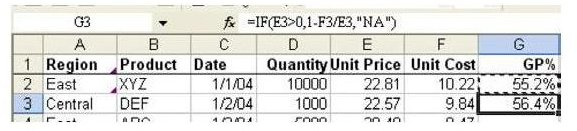So, the solution to the problem is simply to copy the formula down to all the other rows. A shortcut for doing this is to select the cell and then double-click the Fill handle to copy the formula down to all rows with values in the adjacent column.

Additional Details: Relative references will move in all four directions. In Fig. 169, if you copy the formula in cell F7 to E6, the referenced cell will change from D3 to C2.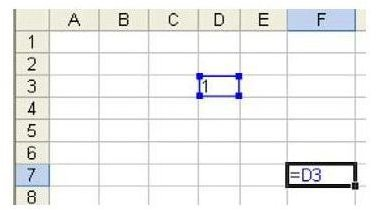In Fig. 170, you can see how the formula copied from F7 to E6:G8 will change.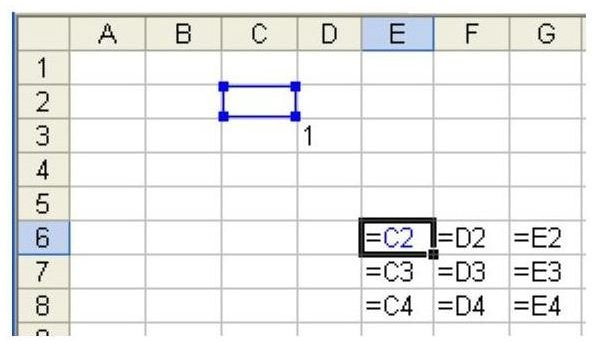Hint: Fig. 170 was shot in Show Formula mode. To enter Show Formula mode, hit Ctrl+~. To toggle back to regular mode, hit Ctrl+~ again.

Gotcha: It is possible to copy a formula so that it will point to a cell that does not exist.? As shown in Fig. 171, what would happen if you copied C4 to B3?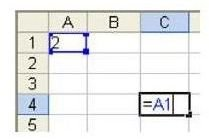The reference to A1 would have to point to the cell one row above and one column to the left of A1. This cell does not exist, so Excel will return a #REF error, as shown in Fig. 172.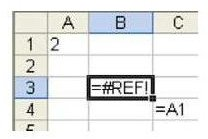Summary: The “miracle” of Excel is that you can enter a formula in one place and copy it to many other places and it will still work. This is because a regular cell reference, such as B1, is a relative reference.

Note: If your formula contains an absolute, or fixed, reference, you may need to modify it slightly before you can copy it to other cells. To find out how to do this, see Microsoft Excel: Copy a Formula While Keeping One Reference Fixed.